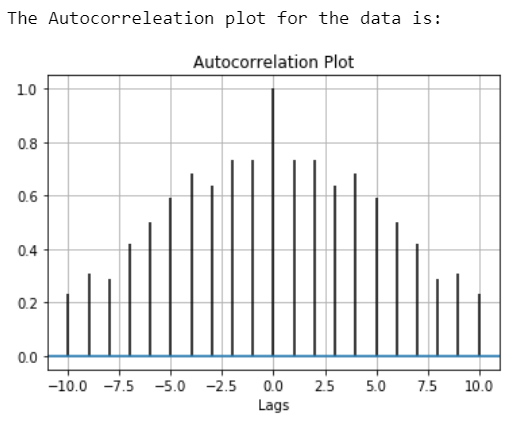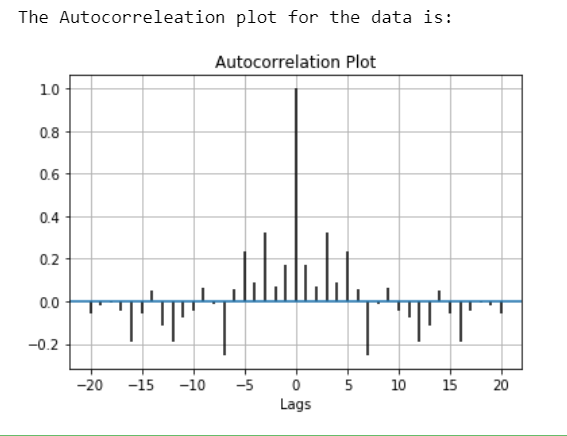# Autocorrelation plot using Matplotlib

Autocorrelation plots are a commonly used tool for checking randomness in a data set. This randomness is ascertained by computing autocorrelations for data values at varying time lags.

Characterstics Of Autocorrelation Plot :

• It measures a set of current values against a set of past values and finds whether they correlate.
• It is the correlation of one-time series data to another time series data which has a time lag.
• It varies from +1 to -1.
• An autocorrelation of +1 indicates that if the time series one increases in value the time series 2 also increases in proportion to the change in time series 1.
• An autocorrelation of -1 indicates that if the time series one increases in value the time series 2 decreases in proportion to the change in time series 1.

Application of Autocorreation:

• Pattern recognition.
• Signal detection.
• Signal processing.
• Estimating pitch.
• Technical analysis of stocks.

## Plotting the Autocorrelation Plot

To plot the Autocorrelation Plot we can use matplotlib and plot it easily by using `matplotlib.pyplot.acorr()` function.

Syntax: matplotlib.pyplot.acorr(x, *, data=None, **kwargs)

parameters:

• ‘x’ : This parameter is a sequence of scalar.
• ‘detrend’ : This parameter is an optional parameter. Its default value is mlab.detrend_none.
• ‘normed’ : This parameter is also an optional parameter and contains the bool value. Its default value is True.
• ‘usevlines’ : This parameter is also an optional parameter and contains the bool value. Its default value is True.
• ‘maxlags’ : This parameter is also an optional parameter and contains the integer value. Its default value is 10.
• ‘linestyle’ : This parameter is also an optional parameter and used for plotting the data points, only when usevlines is False.
• ‘marker’ : This parameter is also an optional parameter and contains the string. Its default value is ‘o’.

Returns: (lags, c, line, b)
Where:

• lags are a length 2`maxlags+1 lag vector.
• c is the 2`maxlags+1 auto correlation vectorI.
• line is a Line2D instance returned by plot.
• b is the x-axis.

Example 1:

 `# Importing the libraries. ` `import` `matplotlib.pyplot as plt  ` `import` `numpy as np  ` `   `  `# Data for which we plot Autocorreleation. ` `data ``=` `np.array([``12.0``, ``24.0``, ``7.``, ``20.0``, ` `                 ``7.0``, ``22.0``, ``18.0``,``22.0``, ` `                 ``6.0``, ``7.0``, ``20.0``, ``13.0``, ` `                 ``8.0``, ``5.0``, ``8``]) ` `   `  `# Adding plot title. ` `plt.title(``"Autocorrelation Plot"``)  ` ` `  `# Providing x-axis name. ` `plt.xlabel(``"Lags"``)  ` ` `  `# Plotting the Autocorreleation plot. ` `plt.acorr(data, maxlags ``=` `10``)  ` ` `  `# Displaying the plot. ` `print``(``"The Autocorreleation plot for the data is:"``) ` `plt.grid(``True``) ` ` `  `plt.show()  `

Output:Example 2:

 `# Importing the libraries. ` `import` `matplotlib.pyplot as plt  ` `import` `numpy as np  ` `   `  `# Setting up the rondom seed for  ` `# fixing the random state. ` `np.random.seed(``42``)  ` `   `  `# Creating some random data. ` `data ``=` `np.random.randn(``25``)  ` `   `  `# Adding plot title. ` `plt.title(``"Autocorrelation Plot"``) ` ` `  `# Providing x-axis name. ` `plt.xlabel(``"Lags"``) ` ` `  `# Plotting the Autocorreleation plot. ` `plt.acorr(data, maxlags ``=` `20``)  ` ` `  `# Displaying the plot. ` `print``(``"The Autocorreleation plot for the data is:"``) ` `plt.grid(``True``) ` ` `  `plt.show()  `

Output:My Personal Notes arrow_drop_upIf you like GeeksforGeeks and would like to contribute, you can also write an article using contribute.geeksforgeeks.org or mail your article to contribute@geeksforgeeks.org. See your article appearing on the GeeksforGeeks main page and help other Geeks.

Please Improve this article if you find anything incorrect by clicking on the "Improve Article" button below.

Article Tags :

Be the First to upvote.

Please write to us at contribute@geeksforgeeks.org to report any issue with the above content.